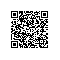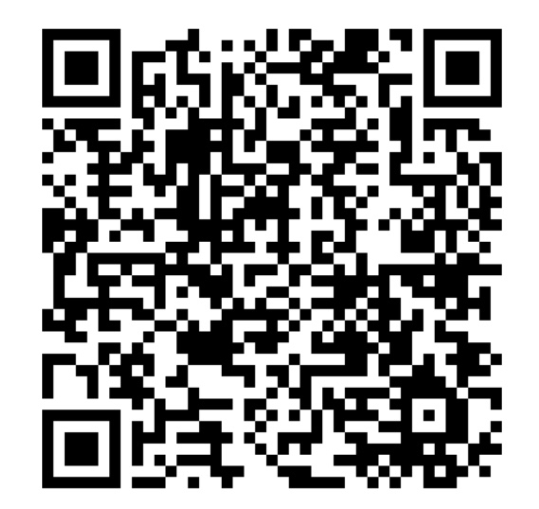# mongoDB geohash index - use prefix base 2bit spilted 2D

http://docs.mongodb.org/manual/core/geospatial-indexes/#geospatial-indexes-geohash

## Calculation of Geohash Values for 2d Indexes

When you create a geospatial index on legacy coordinate pairs, MongoDB computes geohash values for the coordinate pairs within the specified location range and then indexes the geohash values.

To calculate a geohash value, recursively divide a two-dimensional map into quadrants. Then assign each quadrant a two-bit value. For example, a two-bit representation of four quadrants would be:

01  11

00  10


These two-bit values (00, 01, 10, and 11) represent each of the quadrants and all points within each quadrant. For a geohash with two bits of resolution, all points in the bottom left quadrant would have a geohash of 00. The top left quadrant would have the geohash of 01. The bottom right and top right would have a geohash of 10 and 11, respectively.

To provide additional precision, continue dividing each quadrant into sub-quadrants. Each sub-quadrant would have the geohash value of the containing quadrant concatenated with the value of the sub-quadrant. The geohash for the upper-right quadrant is 11, and the geohash for the sub-quadrants would be (clockwise from the top left): 1101, 1111, 1110, and 1100, respectively.使用钉钉扫一扫加入圈子
+ 订阅